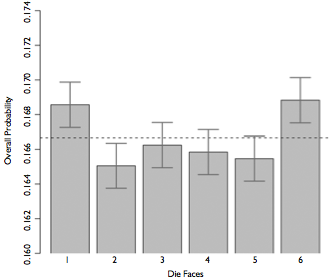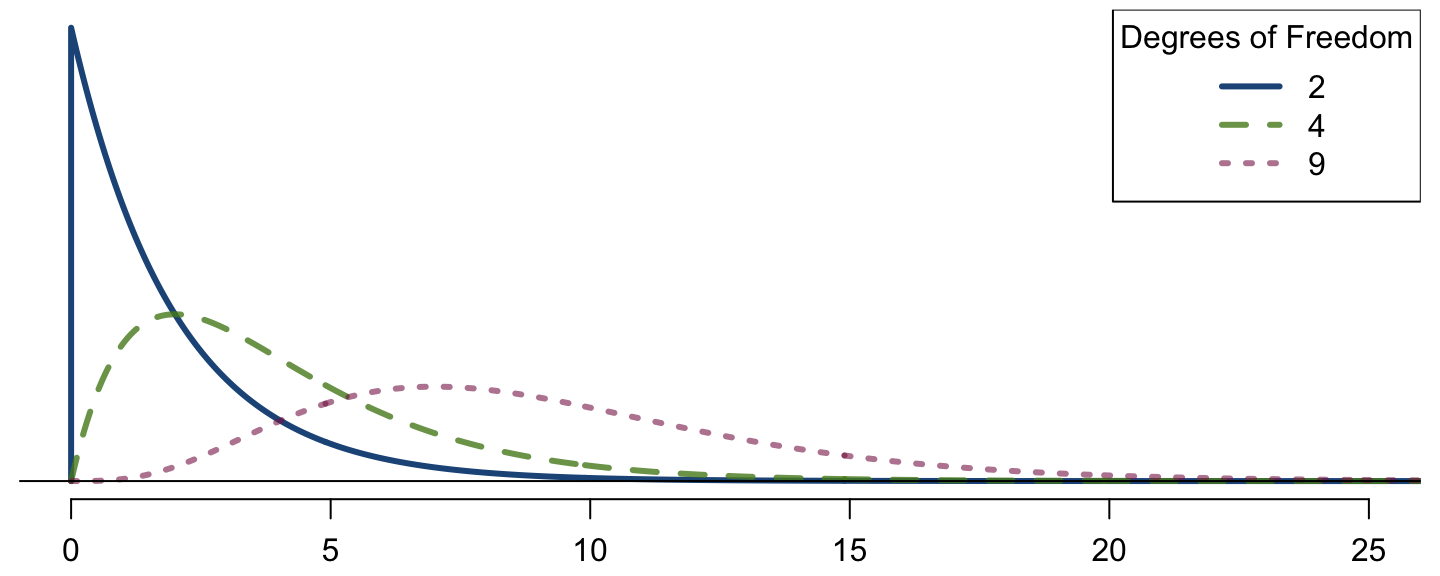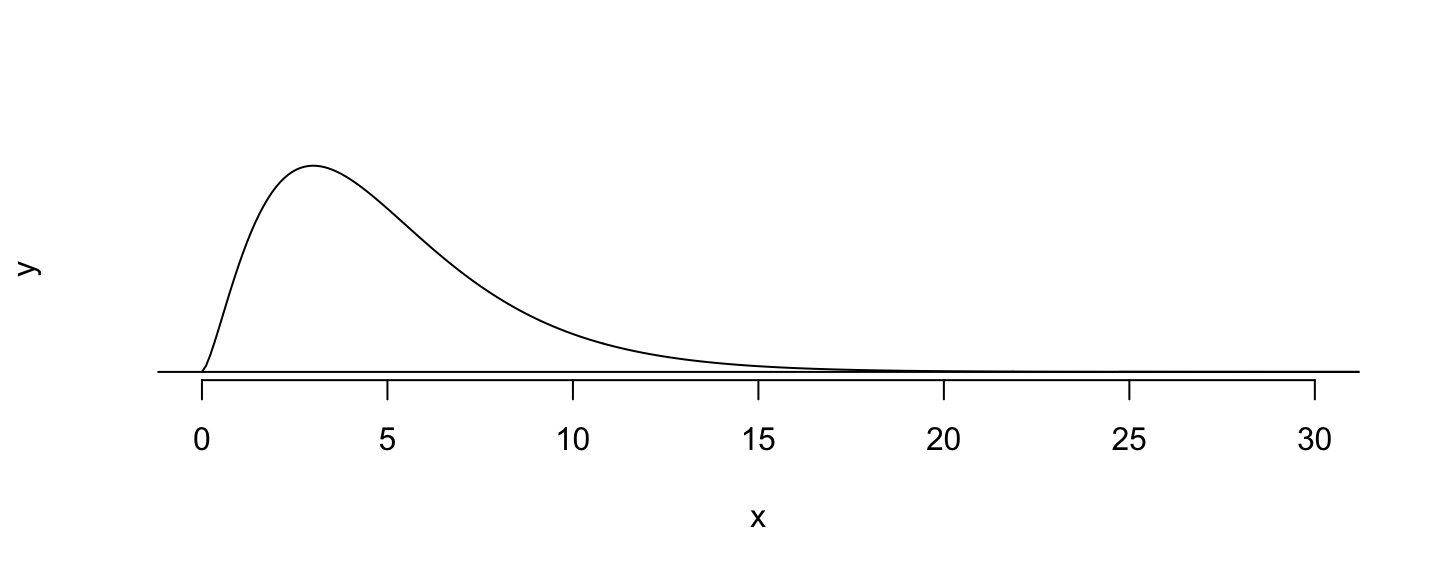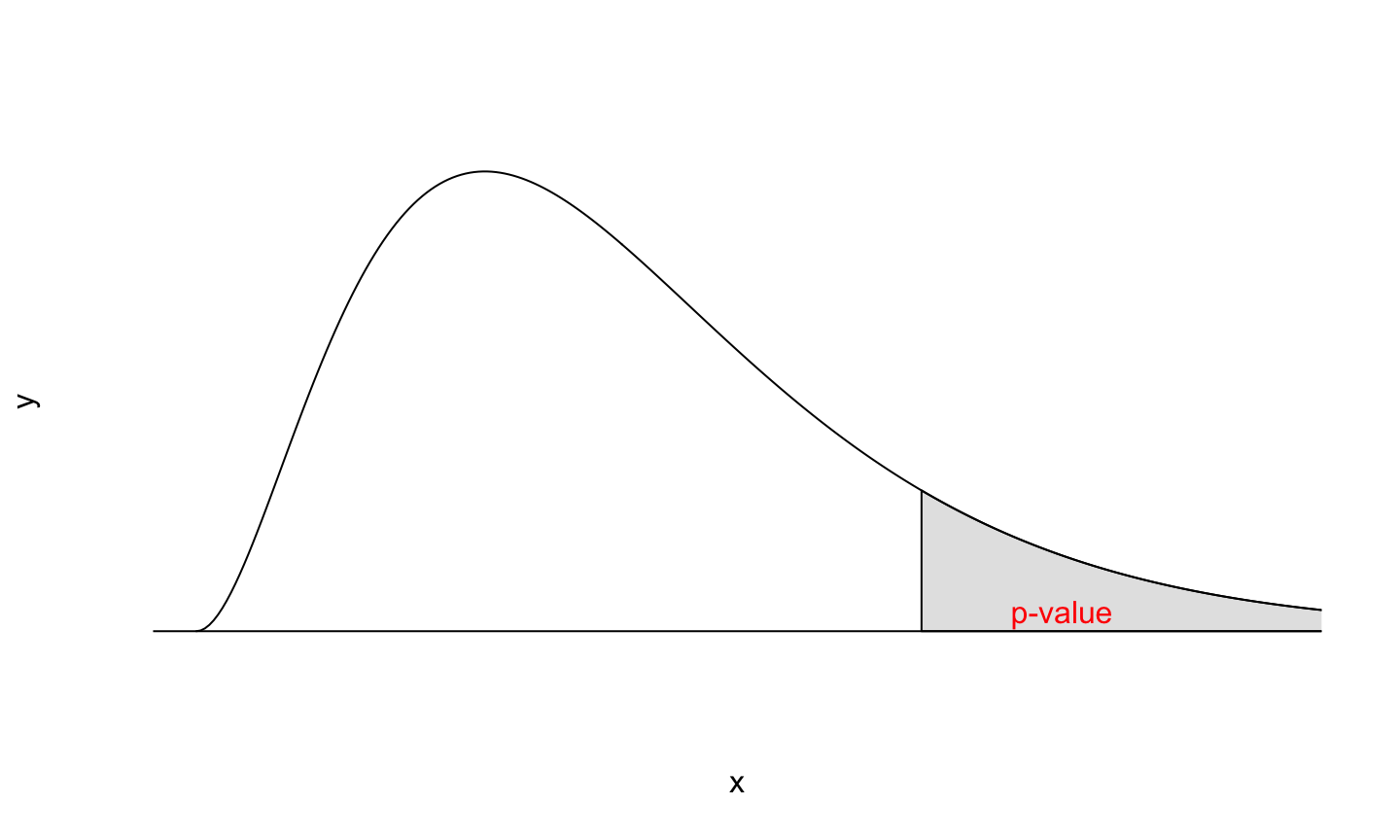March 11, 2020

## Announcements

• We switch to Zoom next week. Look for the link on the Meetups page. I will also post it in Slack.

## Example

Two scientists want to know if a certain drug is effective against high blood pressure. The first scientist wants to give the drug to 1,000 people with high blood pressure and see how many of them experience lower blood pressure levels. The second scientist wants to give the drug to 500 people with high blood pressure, and not give the drug to another 500 people with high blood pressure, and see how many in both groups experience lower blood pressure levels. Which is the better way to test this drug?

• 500 get the drug, 500 don’t

## Survey of Americans

The GSS asks the same question, below is the distribution of responses from the 2010 survey:

Response n
All 1000 get the drug 99
500 get the drug 500 don’t 571
Total 670

## Parameter of Interest

• Parameter of interest: Proportion of all Americans who have good intuition about experimental design.
$p(population\; proportion)$

• Point estimate: Proportion of sampled Americans who have good intuition about experimental design.
$\hat{p}(sample\; proportion)$

## Inference for a proportion

What percent of all Americans have good intuition about experimental design (i.e. would answer “500 get the drug 500 don’t?”

• Using a confidence interval $point\; estimate \pm ME$

• We know that ME = critical value x standard error of the point estimate. $SE_{\hat{p}} = \sqrt{\frac{p(1-p)}{n}}$

## Central limit theoreom for proportions

Sample proportions will be nearly normally distributed with mean equal to the population mean, p, and standard error equal to $$\sqrt{\frac{p(1-p)}{n}}$$.

$\hat { p } \sim N\left( mean=p,SE=\sqrt { \frac { p(1-p) }{ n } } \right)$

This is true given the following conditions:

• independent observations
• at least 10 successes and 10 failures

## Back to the Survey

• 571 out of 670 (85%) of Americans answered the question on experimental design correctly.
• Estimate (using a 95% confidence interval) the proportion of all Americans who have good intuition about experimental design?

Given: $$n = 670$$, $$\hat{p} = 0.85$$.

Conditions:

1. Independence: The sample is random, and 670 < 10% of all Americans, therefore we can assume that one respondent’s response is independent of another.

2. Success-failure: 571 people answered correctly (successes) and 99 answered incorrectly (failures), both are greater than 10.

## Calculating Confidence Interval

Given: $$n = 670$$, $$\hat{p} = 0.85$$.

$0.85 \pm 1.96 \sqrt{\frac{0.85 \times 0.15}{670}} = \left(0.82,\; 0.88\right)$

We are 95% confidence the true proportion of Americans that have a good intuition about experimental designs is betwee 82% and 88%.

## How many should we sample?

Suppose you want a 3% margin of error, how many people would you have to survey?

Use $$\hat{p} = 0.5$$

• If you don’t know any better, 50-50 is a good guess
• $$\hat{p} = 0.5$$ gives the most conservative estimate - highest possible sample size

$0.03 = 1.96 \times \sqrt{\frac{0.5 \times 0.5}{n}}$ $0.03^2 = 1.96^2 \times \frac{0.5 \times 0.5}{n}$ $n \approx 1,068$

## Example: Two Proportions

Scientists predict that global warming may have big effects on the polar regions within the next 100 years. One of the possible effects is that the northern ice cap may completely melt. Would this bother you a great deal, some, a little, or not at all if it actually happened?

Response GSS Duke
A great deal 454 69
Some 124 40
A little 52 4
Not at all 50 2
Total 680 105

## Parameter and Point Estimate

Parameter of interest: Difference between the proportions of all Duke students and all Americans who would be bothered a great deal by the northern ice cap completely melting.

$p_{Duke} - p_{US}$

Point estimate: Difference between the proportions of sampled Duke students and sampled Americans who would be bothered a great deal by the northern ice cap completely melting.

$\hat{p}_{Duke} - \hat{p}_{US}$

## Everything else is the same…

• CI: $$point\; estimate \pm margin\; of\; error$$
• HT: $$Z = \frac{point\; estimate - null\; value}{SE}$$

Standard error of the difference between two sample proportions

$SE_{\hat{p}_1 - \hat{p}_2} = \sqrt{ \frac{p_1\left(1 - p_1\right)}{n_1} + \frac{p_2\left(1 - p_2\right)}{n_2} }$

Conditions:

1. Independence within groups: The US group is sampled randomly and we’re assuming that the Duke group represents a random sample as well. $$n_{Duke} < 10\%$$ of all Duke students and $$680 < 10\%$$ of all Americans.
2. Independence between groups: The sampled Duke students and the US residents are independent of each other.
3. Success-failure: At least 10 observed successes and 10 observed failures in the two groups.

Construct a 95% confidence interval for the difference between the proportions of Duke students and Americans who would be bothered a great deal by the melting of the northern ice cap ($$p_{Duke} - p_{US}$$).

Data Duke US
A great deal 69 454
Not a great deal 36 226
Total 105 680
$$\hat{p}$$ 0.657 0.668

$\left(\hat{p}_{Duke} - \hat{p}_{US}\right) \pm z* \times \sqrt{ \frac{p_{Duke}\left(1 - p_{Duke}\right)}{n_{Duke}} + \frac{p_{US}\left(1 - p_{US}\right)}{n_{US}} }$

$(0.657 - 0.668) \pm 1.96 \times \sqrt{\frac{0.657 \times 0.343}{105} + \frac{0.668 \times 0.332}{680}} = \left(-0.108,\; 0.086\right)$

## Weldon’s dice

• Walter Frank Raphael Weldon (1860 - 1906), was an English evolutionary biologist and a founder of biometry. He was the joint founding editor of Biometrika, with Francis Galton and Karl Pearson.
• In 1894, he rolled 12 dice 26,306 times, and recorded the number of 5s or 6s (which he considered to be a success).
• It was observed that 5s or 6s occurred more often than expected, and Pearson hypothesized that this was probably due to the construction of the dice. Most inexpensive dice have hollowed-out pips, and since opposite sides add to 7, the face with 6 pips is lighter than its opposing face, which has only 1 pip.

## Labby’s dice

In 2009, Zacariah Labby (U of Chicago), repeated Weldon’s experiment using a homemade dice-throwing, pip counting machine. http:// www.youtube.com/ watch?v= 95EErdouO2w

• The rolling-imaging process took about 20 seconds per roll.## Summarizing Labby’s results

The table below shows the observed and expected counts from Labby’s experiment.

Outcome Observed Expected
1 53,222 52,612
2 52,118 52,612
3 52,465 52,612
4 52,338 52,612
5 52,244 52,612
6 53,285 52,612
Total 315,672 315,672

## Setting the hypotheses

Do these data provide convincing evidence of an inconsistency between the observed and expected counts?

• $$H_0$$: There is no inconsistency between the observed and the expected counts. The observed counts follow the same distribution as the expected counts.

• $$H_A$$: There is an inconsistency between the observed and the expected counts. The observed counts do not follow the same distribution as the expected counts. There is a bias in which side comes up on the roll of a die.

## Evaluating the hypotheses

• To evaluate these hypotheses, we quantify how different the observed counts are from the expected counts.
• Large deviations from what would be expected based on sampling variation (chance) alone provide strong evidence for the alternative hypothesis.
• This is called a test since we’re evaluating how well the observed data fit the expected distribution.

## Anatomy of a test statistic

• The general form of a test statistic is:

$\frac{\text{point estimate} - \text{null value}}{\text{SE of point estimate}}$

• This construction is based on
1. identifying the difference between a point estimate and an expected value if the null hypothesis was true, and
2. standardizing that difference using the standard error of the point estimate.
• These two ideas will help in the construction of an appropriate test statistic for count data.

## Chi-Squared

When dealing with counts and investigating how far the observed counts are from the expected counts, we use a new test statistic called the chi-square ($$\chi^2$$) statistic.

$\chi^2 = \sum_{i = 1}^k \frac{(O - E)^2}{E} \qquad \text{where k = total number of cells}$

Outcome Observed Expected $$\frac{(O - E)^2}{E}$$
1 53,222 52,612 $$\frac{(53,222 - 52,612)^2}{52,612} = 7.07$$
2 52,118 52,612 $$\frac{(52,118 - 52,612)^2}{52,612} = 4.64$$
3 52,465 52,612 $$\frac{(52,465 - 52,612)^2}{52,612} = 0.41$$
4 52,338 52,612 $$\frac{(52,338 - 52,612)^2}{52,612} = 1.43$$
5 52,244 52,612 $$\frac{(52,244 - 52,612)^2}{52,612} = 2.57$$
6 53,285 52,612 $$\frac{(53,285 - 52,612)^2}{52,612} = 8.61$$
Total 315,672 315,672 24.73

## Chi-Squared Distribution

Squaring the difference between the observed and the expected outcome does two things:

• Any standardized difference that is squared will now be positive.
• Differences that already looked unusual will become much larger after being squared.

In order to determine if the $$\chi^2$$ statistic we calculated is considered unusually high or not we need to first describe its distribution.

• The chi-square distribution has just one parameter called degrees of freedom (df), which influences the shape, center, and spread of the distribution.## Degrees of freedom for a goodness of fit test

When conducting a goodness of fit test to evaluate how well the observed data follow an expected distribution, the degrees of freedom are calculated as the number of cells ($$k$$) minus 1.

$df = k - 1$

For dice outcomes, $$k = 6$$, therefore $$df = 6 - 1 = 5$$p-value = $$P(\chi^2_{df = 5} > 24.67)$$ is less than 0.001

## Turns out…

• The 1-6 axis is consistently shorter than the other two (2-5 and 3-4), thereby supporting the hypothesis that the faces with one and six pips are larger than the other faces.
• Pearson’s claim that 5s and 6s appear more often due to the carved-out pips is not supported by these data.
• Dice used in casinos have flush faces, where the pips are filled in with a plastic of the same density as the surrounding material and are precisely balanced.

## Recap: p-value for a chi-square test

• The p-value for a chi-square test is defined as the tail area above the calculated test statistic.

• This is because the test statistic is always positive, and a higher test statistic means a stronger deviation from the null hypothesis.